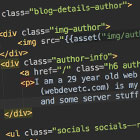## PHP: How to find the intersection (same values) from two arrays?

If you have two (or more) arrays with various values, how do you find the values from the 1st array that are also in the 2nd (and others) array? Use the intersect functions.

PHP has a nice collection of array functions - one of which is `array_intersect`. You can use it to solve this problem.

`       \$array1 = ['apple','banana','grape','orange'];\$array2 = ['cake', 'apple', 'toast', ]; \$vals_in_both_arrays =  array_intersect(\$array1,\$array2);`

Which outputs an array with the single item in both arrays: `['apple']`.

### What if you need to compare the keys and values?

Then use `array_intersect_assoc()` which will compare both keys and values.

` \$array1 = ["aa" => "a",                    "bb" => "b",                    "cc" => "c",                    "dd" => "d"                ];         \$array2 = [             "dd" =>"d", // same as above            "foo" => "bar",            "bb" => "not_b",            "cc" => "c", //same as above            "aa" => "not_a",              ];          \$same = (array_intersect_assoc(\$array1,\$array2));`
```array:2 [
"cc" => "c"
"dd" => "d"
]```

If you just want to compare the keys, then use `array_intersect_keys()`.webdevetc

I am a 29 year old backend web developer from London, mostly focusing on PHP and Laravel lately. This (webdevetc.com) is my blog where I write about some web development topics (PHP, Laravel, Javascript, and some server stuff). contact me here.

### More...

##### Or see other languages/frameworks:
PHP Laravel Composer Apache CentOS and Linux Stuff WordPress General Webdev and Programming Stuff JavaScript